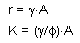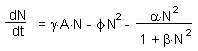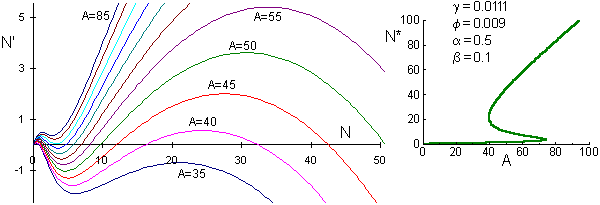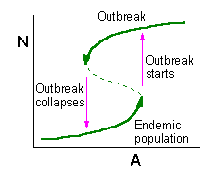## Catastrophe theory

Catastrophe theory was very fashionable in 70-s and 80-s. Rene Thom was one of its spiritual leaders. This theory originated from qualitative solution of differential equations and it has nothing in common with Apocalypse or UFO.

Catastrophe means the loss of stability in a dynamic system. The major method of this theory is sorting dynamic variables into slow and fast. Then stability features of fast variables may change slowly due to dynamics of slow variables.

The theory of catastrophes was applied to the spruce budworm (Choristoneura fumiferana) (Casti 1982, Ecol. Modell., 14: 293-300). We will use the model which was considered in the previous section and modify it by adding a slow variable: the average age of trees in the stand.

The performance of spruce budworm populations is better in mature spruce stands than in young stands. Thus, we will assume that the intrinsic rate of increase (r) and carrying capacity (K) both increase with the age of host trees:where A is the average age of trees in a stand. Now the model is represented by the equation:The first term is the logistic model, and the second term describes mortality caused by generalist predators which have a type III functional response. Equilibrium points can be found by solving this equation with the left part set to zero (dN/dt = 0):The left graph shows phase plots for various forest ages from A = 35 to A = 85, and the right graph shows equilibrium points (where the derivative is equal to 0). Only one non-zero equilibrium exists if N<38 or N>74. If 40<A<74, then there are 2 two stable equilibria separated by one unstable equilibrium. Equilibrium line folds.

The age of trees continue increasing with time. Age can be considered as a "slow" variable as compared to population density which is a "fast" variable. Dynamics of the system can be explained using the graph:Fast processes are vertical arrows; slow processes are thick arrows. Slow processes go along stable lines until it ends, then there is a fast "jump" to another stable line.

Direction of slow processes. When the density of spruce budworm is low, then there is little mortality of trees and the average age of trees increases. Thus, the slow process at the lower branch of stable budworm density is directed to the right (increasing of stand age). The upper branch of stable budworm density corresponds to outbreak populations. Old trees are more susceptible to defoliation and they die first. Thus, the mean age of defoliated stand decreases, and the slow process at the upper branch goes backwards.Population dynamics can be described as a limit cycle that includes 2 periods of slow change and 2 periods of fast change. Transition to a fast process is a catastrophe. This model is built in Excel:

We can add stochastic fluctuations due to weather or other factors. As the age of trees increases, the domain of attraction of the endemic (=low-density) equilibrium becomes smaller. As a result, the probability of outbreak increases with increasing forest age. If an outbreak occurs in a young forest stand, then it is possible to suppress the population and return it back to the area of stability. But if the stand is old, then the endemic equilibrium has a very narrow domain of attraction, and thus, the probability of an outbreak is very high. Finally, the lower equilibrium disappears and it is no longer possible to avoid an outbreak by suppressing budworm population.

The model suggests to reduce forest age by cutting oldest trees. This will move the system back into the stable area.

Alexei Sharov 04/17/97